### Lewis Carroll's Five Liar Problem

[From Symbolic Logic by Lewis Carroll, 2nd edition, London:1896] 

There are 5 friends, A, B, C, D and E, each of whom makes 2 assertions, so that it is possible for him to tell 2 Truths, or a Truth and Lie, or 2 Lies.

A says:
"Either B or D tells a Truth and a Lie.
Either C or E tells 2 Lies."
B says:
"Either A or C tells a Truth and a Lie.
Either D tells 2 Lies or E tells 2 Truths."
C says:
"Either A or D tells 2 Truths.
Either B tells a Truth and a Lie or E tells 2 Lies."
D says:
"Either A or E tells 2 Lies.
Either B tells 2 Lies or C tells 2 Truths."
E says:
"Either A or B tells 2 Truths.
Either C or D tells a Truth and a Lie."

What is the condition, as to truth-telling and lying, of each of these five painfully-candid friends?

[It is assumed that 'Either p or q' means that either p is the case and q is not; or q is the case and p is not.]

There are ten assertions in the problem, each of which may be true or false. Our task is to assign truth-values to the assertions in a consistent fashion. The difficulty is as follows: taking (for example) A's first assertion, its truth (or otherwise) is tied in with the truth (or otherwise) of both of B's assertions and both of D's assertions. The same goes for each of the remaining assertions. As a first step towards a solution, we need to find an economical way of representing the 'tyings in' or relationships between the truth-values of the assertions.

There are 210=1024 ways of assigning truth-values to ten assertions. A brute force approach to the problem would be to try out each of these assignments in turn, in each case looking to see if the ten relationships were satisfied. 10,240 items to check. Even though we aren't actually going to do this, let's think about what we would have to check if we were going to take this approach.

Consider this: there is A's first assertion - 'Either B or D tells a truth and a lie' - and there is the 'truth or otherwise' of this assertion. These are two different things. We can represent the 'truth or otherwise' of the assertion by what is called (by computer programming languages, for example) a Boolean variable. Let's call the variable a: it can take two values, true and false. Only we're going to use a sort of code for true and false: true will be represented by 'no mark', _ ; while false will be represented by a mark O.

So a=_ means 'A's first assertion is true'; a=O means 'A's first assertion is false'.

In just the same way, let us assign Boolean variables to each of the remaining nine assertions, as follows: to A's second assertion, f; to B's two assertions, b and g; to C's, c and h; to D's, d and i; to E's, e and j.

The assertion 'B tells a truth and a lie' can then be translated as

either b=_ and g=O; or b=O and g=_ .

This statement may be translated into an even more concise form, if we avail ourselves of the equivalence expression (see Development of the Equivalence Expression). Our more concise statement is simply

[b|(g)] = _ ;

this is shorthand for

(bg)((b)(g)) = _ .

Taking this a stage further, the assertion 'Either B or D tells a Truth and a Lie' can be converted, firstly, into the form

either [b|(g)]=_ and [d|(i)]=O; or [b|(g)]=O and [d|(i)]=_ ,

and then, more concisely, into

[ [b|(g)] | ([d|(i)]) ] = _ .

Now for the 'tying in'. A's first assertion is true if and only if the statement just made is true. Since the only values that may be taken by the expression on the left-hand side of that statement are _ and O, the 'tying in' may be expressed by the following equation:

a = [ [b|(g)] | ([d|(i)]) ] ,

or,

[a| [ [b|(g)] | ([d|(i)]) ] ] = _ .

The advantage of this last way of putting it may not be immediately apparent (but will emerge as we go on).

For any assignment of values to the ten variables, this equation is one of the things we have to check. We may go on to treat A's second statement in a similar fashion. The value of the assertion 'C tells two lies' is

(c)(h);

for this expression takes the value _ if and only if both c and h take the value O (=false). The value of the assertion 'Either C or E (but not both) tells 2 lies' is

([(c)(h)|(e)(j)]);

and f is tied in by the equation

[f| ([(c)(h)|(e)(j)]) ] = _ .

Eight more equations may be derived in a similar manner:

[b| ([ [a|(f)] | [c|(h)] ]) ] = _
[g| ([ (d)(i)|ej ]) ] = _
[c| ([af|di]) ] = _
[h| ([ [b|(g)] | (e)(j) ]) ] = _
[d| ([ (a)(f) | (e)(j) ]) ] = _
[i| ([ (b)(g) | ch ]) ] = _
[e| ([ af|bg ]) ] = _
[j| ([ [c|(h)] | [d|(i)] ]) = _ .

We may now simplify each of these expressions by deploying the technology of the multiple equivalence expressions (see Development of the Equivalence Expression):

[a|b|g|d|(i)] = _
[(f)|(c)(h)|(e)(j)] = _
[b|a|f|c|(h)] = _
[(g)|(d)(i)|ej ] = _
[(c)|af|di] = _
[h|b|g|(e)(j)] = _
[(d)|(a)(f)|(e)(j)] = _
[(i)|(b)(g)|ch] = _
[(e)|af|bg] = _
[j|c|h|d|(i)] = _ .

The condition that all ten of these expressions are void (i.e. have value _ ) can be re-expressed as the condition that the expression E that is their product is void. Here is a pictorial representation of E: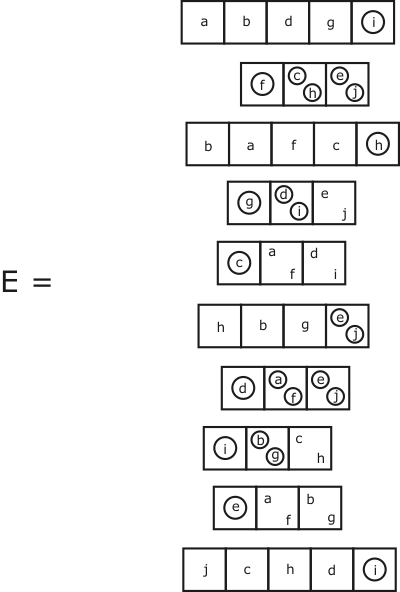The problem is to find the values of the variables (if any) that make E equivalent to no mark. You must admit that it would be hard to find a more parsimonious representation of the conditions of the problem!

We now proceed to simplify E, as far as possible, using the methods of the CL-calculus. The idea is to avoid the wearisome task of going through all the 1024 possible assignments to the variables. To this end, we first apply the 'Case Analysis' Lemma, with respect to the letter a. (We chose a just because it happens to come first in the alphabet - and first in the problem!)

E = ((a Ea) ((a) E(a))).

We recall that Ea is the expression that results when every occurrence of a in E is replaced by _ ; E(a) is the expression that results when every occurrence of a in E is replaced by O. So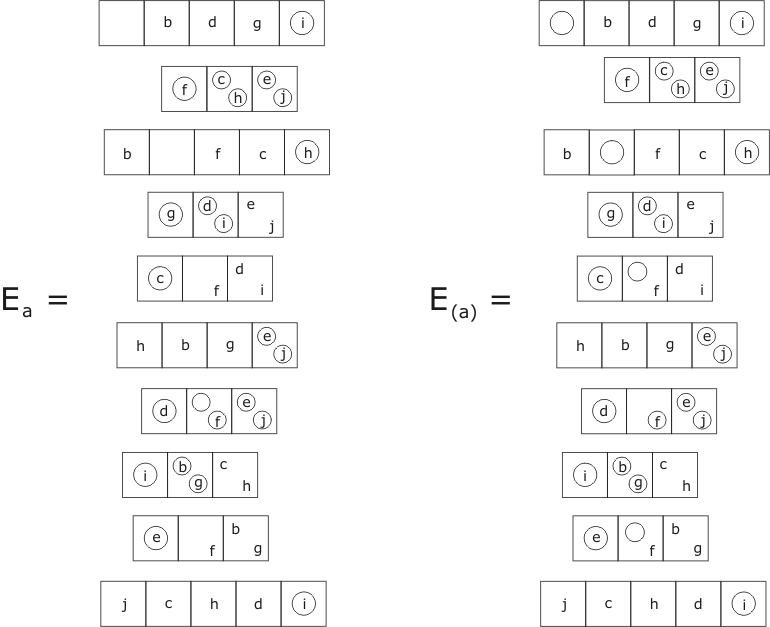Simplifying, using the properties of the multiple equivalence expression, we get: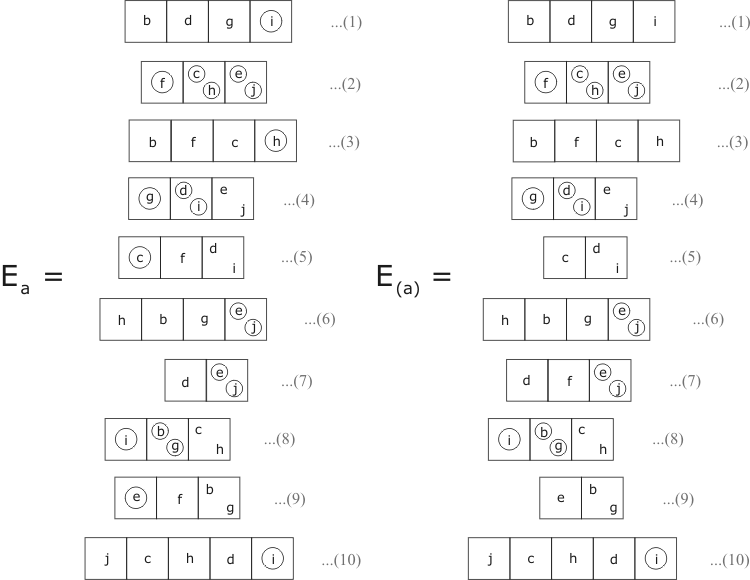From here on we treat Ea and E(a) separately. Taking first Ea, we gradually transform the various factors (identified by the numbers in the last figure) using the other factors as 'catalysts'. Thus, in the first step (below) we transform factor number 6 using factor 7 as catalyst. In the presence of factor 7, (e)(j) may be replaced by d. Then, in the presence of factor number 1, [b|g|d] may be replaced by (i), resulting in the new form of factor 6, namely [h|(i)].

We do the same sort of thing, again and again. In each case we use the latest version of the factor as catalyst, so the steps describe a sort of ongoing evolution of the expression Ea.

The steps marked A and B make use of a couple of lemmas, both of which have the effect of reducing the number of compartments in an equivalence expression by one. These are as follows:

Lemma A:

[a|ab] = (a(b)) .

Proof: use the definition of the equivalence expression:

[a|ab] = (a(ab)) ((a)ab) = (a(b)) (()ab) = (a(b)) ◊

Note the relationship of this lemma to Lemma 4 of Mutual Dominance.

There are many variant forms of Lemma A, obtained by applying the identities

[(a)|b] = ([a|b]) = [a|(b)].

For example,

[a|(a)(b)] = ([(a)|(a)(b)]) = (((a)((b)))) = (a)b.

Lemma B:

[ab|a(b)] = (a) .

Proof: Either transliterate the left-hand side of the equation into a circle and letter expression (as in the proof of Lemma A), or use the Case Analysis Lemma. Replacing b by _, the left-hand side of the equation becomes [a|O] = (a); replacing b by O, the left-hand side of the equation becomes [O|a], also equal to (a). Therefore, by the Case Analysis Lemma, and by each way, the left-hand side of the equation is CL-equivalent to (a) ◊

Here now are the steps involved in the evolution of Ea in the direction of simplicity: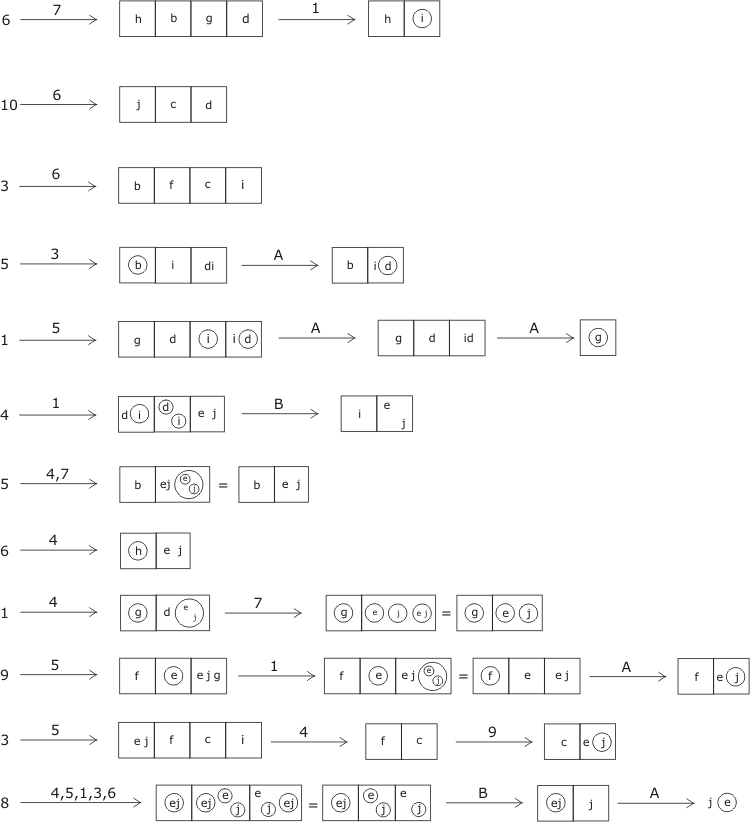With this last step we are really getting somewhere: one of our factors has been reduced to a simple CL-expression (no longer an equivalence expression), which turns it into a still more powerful catalyst. For now the letter j can be uncopied from any other factor, and likewise the expression (e). In other words, in the presence of factor 8, any occurrence of j can be replaced by _ , and any occurrence of e can be replaced by O. Before we exploit this power, we have a bit of tidying up to do. Factors 10 and 2 can both be transformed into the empty expression. Each of them vanishes: they have nothing more to tell us!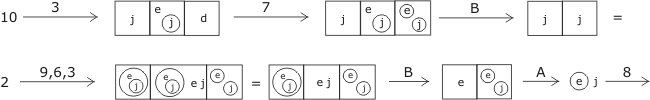So now our expression Ea takes the much-simplified form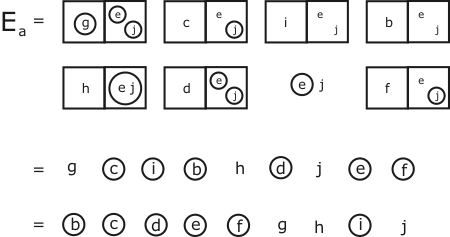This is ultimate simplicity: we can't go any further than this!

We now set about simplifying the second expression, E(a), in a similar way. The sequence of steps which I have found is as follows (you may be able to do better!):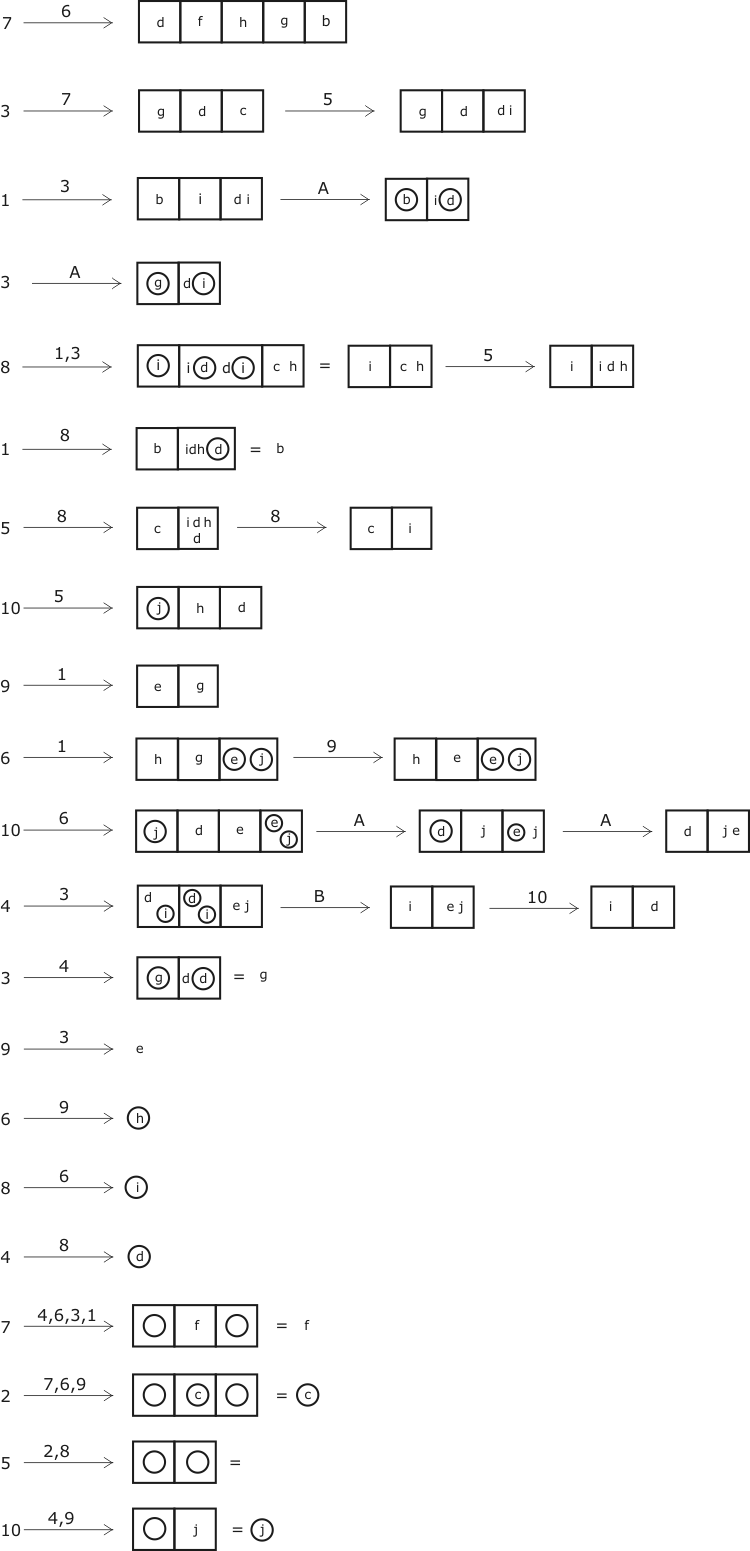Thus E(a) is reduced to the following form: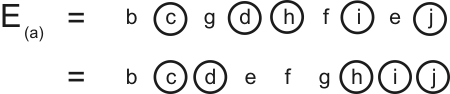Finally, E as a whole has been transformed into

E = ((aEa) ((a)E(a))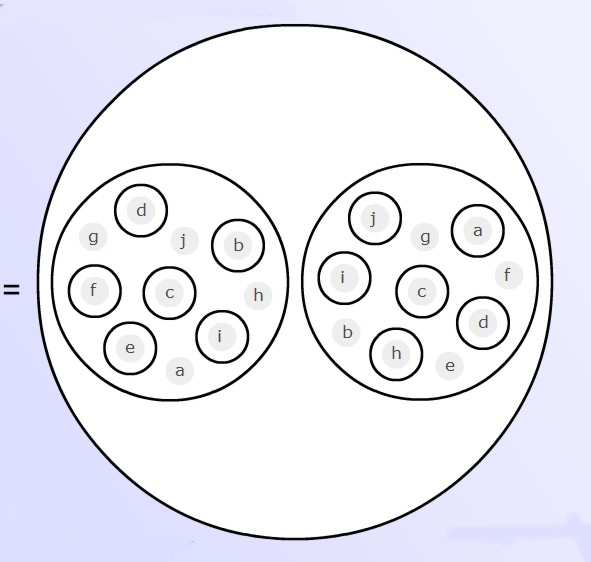It will be seen that it is not impossible for E to be void (i.e. to reduce to the empty expression). It suffices for one or other of the inner expressions to be void. This gives rise to two solutions of the problem:

Solution 1:

A's first assertion is true, their second, false;
B's first assertion is false, their second, true;
C's first assertion is false, their second, true;
both of D's assertions are false;
E's first assertion is false, their second, true.

Thus A, B, C, E each tell a Truth and a Lie;
whereas D tells 2 Lies.

Solution 2:

A's first assertion is false, their second, true;
both of B's assertions are true;
both of C's assertions are false;
both of D's assertions are false;
E's first assertion is true, their second, false.

Thus A and E each tell a Truth and a Lie;
C and D each tell 2 Lies;
B tells 2 Truths.

It will be found that both solutions are indeed consistent with the conditions of the problem.

With hindsight, we can see that our choice of a as the letter to use for 'case analysis' was actually rather fortunate, in that a= _ leads to one of the solutions, a = O to the other. If we had chosen c instead, c = _ would have led to no solution. Indeed, from our final form for E we can see that Ec is equivalent to O (so there is no possibility of making E = _ by taking c = _ ). E(c) on the other hand is a relatively complicated expression; it is unlikely that our method of simplification would have led us to this form. We would probably have got stuck, and would have had to do a second case analysis to get going again.

##### Self-reference

None of the friends makes a statement that refers directly to himself. But if A made a statement about B, and B made a statement about A, taken together the statements would amount to 'indirect' self-reference; and could amount to vicious self-reference. A simple example of the latter would be:

A says: B tells a Truth;
B says: A tells a Lie.

Translated into symbols this says:

[a|[b|_]] [b|[a|O]].

Transforming this 'problem-expression' by the usual rules it turns into:

[a|b] [b|(a)]
= [a|b] [a|(a)]
= [a|b] O
= O .

There are no values for a and b which can make the expression take the value _ . It's value is categorically O, indicating False. The conditions of the problem cannot be satisfied.

This could have happened in the actual problem, but it didn't. Although there is plenty of self-reference in the problem, it is not vicious or necessarily self-contradictory. For this we have the author of the problem to thank.

##### Acknowledgement

This page was inspired by, and is really no more than a footnote to, William Bricken's article Lewis Carroll's Five Liars Puzzle. Bricken's purpose is to show that the problem, once translated into the language of Laws of Form, can be solved automatically by something called the 'LOSP Boolean Minimization Engine'. Mine is to show how it may be done manually by anyone who is sufficiently dedicated. I would describe the task as quite difficult, but not at all boring - not, at least, for anyone who is even a little entranced by the magic of the circle-and-letter form.

Lewis Carroll, Symbolic Logic, e-book available here.